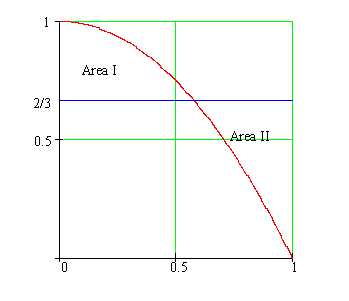Calculus on Demand at Dartmouth College Lecture 11 | Index | Lecture 13 Lecture 12## Resources

Math 3 Course Syllabus
Practice Exams

# Contents

In this lecture we study something called the "Mean Value Theorem." This theorem allows us to take an "at-a-point" concept like the derivative and use it to study the global behavior of a function. We will also learn how to differentiate a function for which we do not have an explicit formula.

### Quick Question

Suppose Area I (above the blue line and below the parabola) equals Area II (above the parabola and below the blue line). What is the total area enclosed by the parabola?### Outline

Outlines for
The Mean Value Theorem
Implicit Differentiation

### Textbook

The Mean Value Theorem
Implicit Differentiation